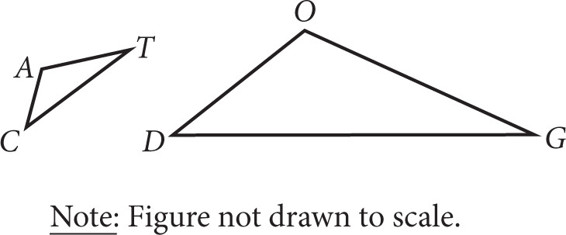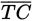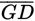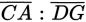# SAT Math Multiple Choice Question 288: Answer and Explanation

### Test Information

Question: 288

3.If ΔCAT shown above is similar to ΔDOG, and the ratio of the length oftois 2:7, which of the following ratios must also be equal to 2:7?

• A.• B. m∠C : m∠D
• C. area of ΔCAT:area of ΔDOG
• D. perimeter of ΔCAT:perimeter of ΔDOG

Explanation:

D

Difficulty: Medium

Category: Additional Topics in Math / Geometry

Strategic Advice: Corresponding sides of similar triangles are proportional, and corresponding angles are congruent.

Getting to the Answer: You can eliminate B immediately because corresponding angles of similar triangles are congruent, so they are always in a 1:1 ratio. You can also eliminate A because side CA does not correspond to side DG (CA corresponds to DO), so you cannot say that they will be in the same ratio. Because the side lengths are proportional, when you add the lengths of all the side lengths (the perimeter), this number will be in the same proportion, so (D) is correct. You can check this by assigning numbers that are in the ratio 2:7 and finding the perimeter of each triangle:

TC = 2 and GD = 7

CA = 4 and DO = 14

AT = 6 and OG = 21

Perimeter of triangle CAT = 2 + 4 + 6 = 12

Perimeter of triangle DOG = 7 + 14 + 21 = 42

12:42 = 2:7